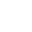Fibonacci Day  Nov 23, 2021It"s a bit of a fib that Fibonacci, the 13th-century Italian math whiz, was the first to sketch out a number sequence in which each number is the sum of the two preceding numbers: 0, 1, 1, 2, 3, 5, 8, 13, and so on forever. In fact, scholars in India described the sequence centuries before Fibonacci, and they probably weren"t the first to figure it out either. But in any case, each November 23—that is, 11/23—we celebrate the infinite series known as the Fibonacci sequence.